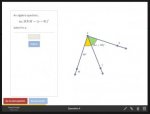# Find for X for m(RXM)=x+81, m(RXT)=2x+32 (Geometry Question)

#### Kal-Don

##### New member
I just started an introduction to geometry course on edx.org and there's an (unexpected) Algebra question to figure out an angle, but I don't really know anything about algebra and how to figure it out. I'm sure it's an easy Algebra problem, but I have no background.

Could someone please show me how to do the work for this question to arrive at the correct answer?

Thank you.Edit:
The above image is small, here's a thumbnail to the full size image, hosted with postimages.Last edited:

#### Jomo

##### Elite Member
I just started an introduction to geometry course on edx.org and there's an (unexpected) Algebra question to figure out an angle, but I don't really know anything about algebra and how to figure it out. I'm sure it's an easy Algebra problem, but I have no background.

Could someone please show me how to do the work for this question to arrive at the correct answer?

Thank you.

View attachment 9396

Edit:
The above image is small, here's a thumbnail to the full size image, hosted with postimages.Well on one hand m<RXM = 2x+32 +40 or 2x+72. On the other hand, it equals x+81. So 2x+72 = x+81. This says that 2 times some unknown number plus 72 equals that same unknown number plus 81. Just think what that number might be. A hint is that it is in between 5 and 15.

#### Kal-Don

##### New member
Thank you, Jomo.

Without knowing how to get the answer I ended up with a few very different answers including -x and 51.

If there's to be more Algebra in the Geometry course I might need to pause Geometry and start the introduction to Algebra course so I can get up to speed.

Thanks, again; much appreciated,
Kalin.

#### Jomo

##### Elite Member
Thank you, Jomo.

Without knowing how to get the answer I ended up with a few very different answers including -x and 51.

If there's to be more Algebra in the Geometry course I might need to pause Geometry and start the introduction to Algebra course so I can get up to speed.

Thanks, again; much appreciated,
Kalin.
x is a place holder for a number, so the answer can not be -x.
You know that 2x+72 = x+81. So replace x with 5. Then 2*5 + 72 =82 while 5+81 = 86. Since 82 $$\displaystyle \neq$$ 86, we know x=5 is not the answer. Try 6...

Also maybe subtract 72 from both sides and get 2x =x+9. That really should help you make a good guess!

#### Kal-Don

##### New member
Thank you for the explanation, Jomo. The process isn't intuitive to me, I'll certainly need to put some time into it as it's been many decades since I was in school.

Thank you for the link, Denis. I appreciate the reference.

I'll mark this topic as solved, then.

I'm off to jump into some beginner Algebra,
Cheers.